Prealgebra

# 3.5Solve Equations Using Integers; The Division Property of Equality

Prealgebra3.5 Solve Equations Using Integers; The Division Property of Equality

### Learning Objectives

By the end of this section, you will be able to:
• Determine whether an integer is a solution of an equation
• Solve equations with integers using the Addition and Subtraction Properties of Equality
• Model the Division Property of Equality
• Solve equations using the Division Property of Equality
• Translate to an equation and solve

### Be Prepared 3.5

Before you get started, take this readiness quiz.

1. $Evaluatex+4whenx=−4.Evaluatex+4whenx=−4.$
If you missed this problem, review Example 3.22.
2. $Solve:y−6=10.Solve:y−6=10.$
If you missed this problem, review Example 2.33.
3. Translate into an algebraic expression $55$ less than $x.x.$
If you missed this problem, review Table 1.3.

### Determine Whether a Number is a Solution of an Equation

In Solve Equations with the Subtraction and Addition Properties of Equality, we saw that a solution of an equation is a value of a variable that makes a true statement when substituted into that equation. In that section, we found solutions that were whole numbers. Now that we’ve worked with integers, we’ll find integer solutions to equations.

The steps we take to determine whether a number is a solution to an equation are the same whether the solution is a whole number or an integer.

### How To

#### How to determine whether a number is a solution to an equation.

1. Step 1. Substitute the number for the variable in the equation.
2. Step 2. Simplify the expressions on both sides of the equation.
3. Step 3.
Determine whether the resulting equation is true.
• If it is true, the number is a solution.
• If it is not true, the number is not a solution.

### Example 3.60

Determine whether each of the following is a solution of $2x−5=−13:2x−5=−13:$

1. $x=4x=4$
2. $x=−4x=−4$
3. $x=−9.x=−9.$

### Try It 3.119

Determine whether each of the following is a solution of $2x−8=−14:2x−8=−14:$

1. $x=−11x=−11$
2. $x=11x=11$
3. $x=−3x=−3$

### Try It 3.120

Determine whether each of the following is a solution of $2y+3=−11:2y+3=−11:$

1. $y=4y=4$
2. $y=−4y=−4$
3. $y=−7y=−7$

### Solve Equations with Integers Using the Addition and Subtraction Properties of Equality

In Solve Equations with the Subtraction and Addition Properties of Equality, we solved equations similar to the two shown here using the Subtraction and Addition Properties of Equality. Now we can use them again with integers.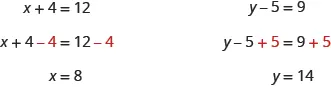When you add or subtract the same quantity from both sides of an equation, you still have equality.

### Properties of Equalities

Subtraction Property of Equality Addition Property of Equality
$For any numbersa,b,c,For any numbersa,b,c,$
$ifa=bthena−c=b−c.ifa=bthena−c=b−c.$
$For any numbersa,b,c,For any numbersa,b,c,$
$ifa=bthena+c=b+c.ifa=bthena+c=b+c.$

### Example 3.61

Solve: $y+9=5.y+9=5.$

### Try It 3.121

Solve:

$y+11=7y+11=7$

### Try It 3.122

Solve:

$y+15=−4y+15=−4$

### Example 3.62

Solve: $a−6=−8a−6=−8$

### Try It 3.123

Solve:

$a−2=−8a−2=−8$

### Try It 3.124

Solve:

$n−4=−8n−4=−8$

### Model the Division Property of Equality

All of the equations we have solved so far have been of the form $x+a=bx+a=b$ or $x−a=b.x−a=b.$ We were able to isolate the variable by adding or subtracting the constant term. Now we’ll see how to solve equations that involve division.

We will model an equation with envelopes and counters in Figure 3.21.

Figure 3.21

Here, there are two identical envelopes that contain the same number of counters. Remember, the left side of the workspace must equal the right side, but the counters on the left side are “hidden” in the envelopes. So how many counters are in each envelope?

To determine the number, separate the counters on the right side into $22$ groups of the same size. So $66$ counters divided into $22$ groups means there must be $33$ counters in each group (since $6÷2=3).6÷2=3).$

What equation models the situation shown in Figure 3.22? There are two envelopes, and each contains $xx$ counters. Together, the two envelopes must contain a total of $66$ counters. So the equation that models the situation is $2x=6.2x=6.$

Figure 3.22

We can divide both sides of the equation by $22$ as we did with the envelopes and counters.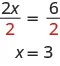We found that each envelope contains $3 counters.3 counters.$ Does this check? We know $2·3=6,2·3=6,$ so it works. Three counters in each of two envelopes does equal six.

Figure 3.23 shows another example.

Figure 3.23

Now we have $33$ identical envelopes and $12 counters.12 counters.$ How many counters are in each envelope? We have to separate the $12 counters12 counters$ into $3 groups.3 groups.$ Since $12÷3=4,12÷3=4,$ there must be $4 counters4 counters$ in each envelope. See Figure 3.24.

Figure 3.24

The equation that models the situation is $3x=12.3x=12.$ We can divide both sides of the equation by $3.3.$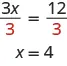Does this check? It does because $3·4=12.3·4=12.$

### Manipulative Mathematics

Doing the Manipulative Mathematics activity “Division Property of Equality” will help you develop a better understanding of how to solve equations using the Division Property of Equality.

### Example 3.63

Write an equation modeled by the envelopes and counters, and then solve it.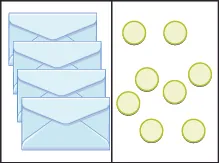### Try It 3.125

Write the equation modeled by the envelopes and counters. Then solve it.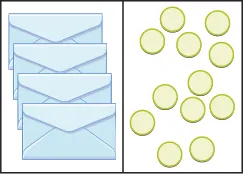### Try It 3.126

Write the equation modeled by the envelopes and counters. Then solve it.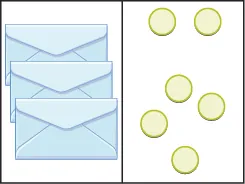### Solve Equations Using the Division Property of Equality

The previous examples lead to the Division Property of Equality. When you divide both sides of an equation by any nonzero number, you still have equality.

### Division Property of Equality

$For any numbers a,b,c,and c≠0, If a=bthen ac=bc. For any numbers a,b,c,and c≠0, If a=bthen ac=bc.$

### Example 3.64

$Solve:7x=−49.Solve:7x=−49.$

### Try It 3.127

Solve:

$8a=568a=56$

### Try It 3.128

Solve:

$11n=12111n=121$

### Example 3.65

Solve: $−3y=63.−3y=63.$

### Try It 3.129

Solve:

$−8p=96−8p=96$

### Try It 3.130

Solve:

$−12m=108−12m=108$

### Translate to an Equation and Solve

In the past several examples, we were given an equation containing a variable. In the next few examples, we’ll have to first translate word sentences into equations with variables and then we will solve the equations.

### Example 3.66

Translate and solve: five more than $xx$ is equal to $−3.−3.$

### Try It 3.131

Translate and solve:

Seven more than $xx$ is equal to $−2−2$.

### Try It 3.132

Translate and solve:

$Eleven more thanyis equal to 2.Eleven more thanyis equal to 2.$

### Example 3.67

Translate and solve: the difference of $nn$ and $66$ is $−10.−10.$

### Try It 3.133

Translate and solve:

The difference of $pp$ and $22$ is $−4−4$.

### Try It 3.134

Translate and solve:

The difference of $qq$ and $77$ is $−3−3$.

### Example 3.68

Translate and solve: the number $108108$ is the product of $−9−9$ and $y.y.$

### Try It 3.135

Translate and solve:

The number $132132$ is the product of $−12−12$ and $yy$.

### Try It 3.136

Translate and solve:

The number $117117$ is the product of $−13−13$ and $zz$.

### Section 3.5 Exercises

#### Practice Makes Perfect

Determine Whether a Number is a Solution of an Equation

In the following exercises, determine whether each number is a solution of the given equation.

285.

$4x−2=64x−2=6$

1. $x=−2x=−2$
2. $x=−1x=−1$
3. $x=2x=2$
286.

$4y−10=−144y−10=−14$

1. $y=−6y=−6$
2. $y=−1y=−1$
3. $y=1y=1$
287.

$9a+27=−639a+27=−63$

1. $a=6a=6$
2. $a=−6a=−6$
3. $a=−10a=−10$
288.

$7c+42=−567c+42=−56$

1. $c=2c=2$
2. $c=−2c=−2$
3. $c=−14c=−14$

Solve Equations Using the Addition and Subtraction Properties of Equality

In the following exercises, solve for the unknown.

289.

$n + 12 = 5 n + 12 = 5$

290.

$m + 16 = 2 m + 16 = 2$

291.

$p + 9 = −8 p + 9 = −8$

292.

$q + 5 = −6 q + 5 = −6$

293.

$u − 3 = −7 u − 3 = −7$

294.

$v − 7 = −8 v − 7 = −8$

295.

$h − 10 = −4 h − 10 = −4$

296.

$k − 9 = −5 k − 9 = −5$

297.

$x + ( −2 ) = −18 x + ( −2 ) = −18$

298.

$y + ( −3 ) = −10 y + ( −3 ) = −10$

299.

$r − ( −5 ) = −9 r − ( −5 ) = −9$

300.

$s − ( −2 ) = −11 s − ( −2 ) = −11$

Model the Division Property of Equality

In the following exercises, write the equation modeled by the envelopes and counters and then solve it.

301.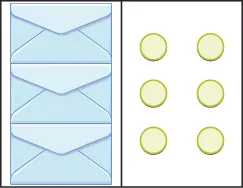302.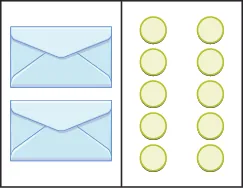303.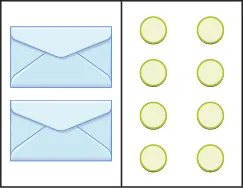304.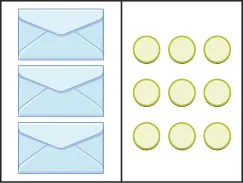Solve Equations Using the Division Property of Equality

In the following exercises, solve each equation using the division property of equality and check the solution.

305.

$5 x = 45 5 x = 45$

306.

$4 p = 64 4 p = 64$

307.

$−7 c = 56 −7 c = 56$

308.

$−9 x = 54 −9 x = 54$

309.

$−14 p = −42 −14 p = −42$

310.

$−8 m = −40 −8 m = −40$

311.

$−120 = 10 q −120 = 10 q$

312.

$−75 = 15 y −75 = 15 y$

313.

$24 x = 480 24 x = 480$

314.

$18 n = 540 18 n = 540$

315.

$−3 z = 0 −3 z = 0$

316.

$4 u = 0 4 u = 0$

Translate to an Equation and Solve

In the following exercises, translate and solve.

317.

Four more than $nn$ is equal to 1.

318.

Nine more than $mm$ is equal to 5.

319.

The sum of eight and $pp$ is $−3−3$.

320.

The sum of two and $qq$ is $−7−7$.

321.

The difference of $aa$ and three is $−14−14$.

322.

The difference of $bb$ and $55$ is $−2−2$.

323.

The number −42 is the product of −7 and $xx$.

324.

The number −54 is the product of −9 and $yy$.

325.

The product of $ff$ and −15 is 75.

326.

The product of $gg$ and −18 is 36.

327.

−6 plus $cc$ is equal to 4.

328.

−2 plus $dd$ is equal to 1.

329.

Nine less than $nn$ is −4.

330.

Thirteen less than $nn$ is $−10−10$.

Mixed Practice

In the following exercises, solve.

331.
1. $x+2=10x+2=10$
2. $2x=102x=10$
332.
1. $y+6=12y+6=12$
2. $6y=126y=12$
333.
1. $−3p=27−3p=27$
2. $p−3=27p−3=27$
334.
1. $−2q=34−2q=34$
2. $q−2=34q−2=34$
335.

$a − 4 = 16 a − 4 = 16$

336.

$b − 1 = 11 b − 1 = 11$

337.

$−8 m = −56 −8 m = −56$

338.

$−6 n = −48 −6 n = −48$

339.

$−39 = u + 13 −39 = u + 13$

340.

$−100 = v + 25 −100 = v + 25$

341.

$11 r = −99 11 r = −99$

342.

$15 s = −300 15 s = −300$

343.

$100 = 20 d 100 = 20 d$

344.

$250 = 25 n 250 = 25 n$

345.

$−49 = x − 7 −49 = x − 7$

346.

$64 = y − 4 64 = y − 4$

#### Everyday Math

347.

Cookie packaging A package of $51 cookies51 cookies$ has $33$ equal rows of cookies. Find the number of cookies in each row, $c,c,$ by solving the equation $3c=51.3c=51.$

348.

Kindergarten class Connie’s kindergarten class has $24 children.24 children.$ She wants them to get into $44$ equal groups. Find the number of children in each group, $g,g,$ by solving the equation $4g=24.4g=24.$

#### Writing Exercises

349.

Is modeling the Division Property of Equality with envelopes and counters helpful to understanding how to solve the equation $3x=15?3x=15?$ Explain why or why not.

350.

Suppose you are using envelopes and counters to model solving the equations $x+4=12x+4=12$ and $4x=12.4x=12.$ Explain how you would solve each equation.

351.

Frida started to solve the equation $−3x=36−3x=36$ by adding $33$ to both sides. Explain why Frida’s method will not solve the equation.

352.

Raoul started to solve the equation $4y=404y=40$ by subtracting $44$ from both sides. Explain why Raoul’s method will not solve the equation.

#### Self Check

After completing the exercises, use this checklist to evaluate your mastery of the objectives of this section.

Overall, after looking at the checklist, do you think you are well-prepared for the next Chapter? Why or why not?

Order a print copy

As an Amazon Associate we earn from qualifying purchases.